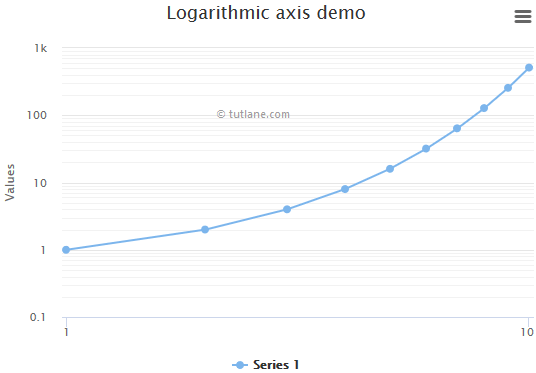# Highcharts Logarithmic Axis Chart

In the previous chapters, we learned how to setup highcharts library and how to create a chart with required configurations using highcharts library in our webpage. Now, we will learn how to create a logarithmic axis chart using highcharts library with examples.

## Highcharts Logarithmic Axis Chart Example

Following is the example of creating a logarithmic axis chart by setting the required logarithmic properties using highcharts library.

<html>

<title>Highcharts Logarithmic Axis Chart</title>

<script src="https://code.jquery.com/jquery-3.4.1.min.js"></script>

<script src="https://code.highcharts.com/highcharts.js"></script>

<script src="https://code.highcharts.com/modules/exporting.js"></script>

<script src="https://code.highcharts.com/modules/export-data.js"></script>

<script type="text/javascript">

\$(function() {

Highcharts.chart('container', {

title: {

text: 'Logarithmic axis demo'

},

xAxis: {

tickInterval: 1,

type: 'logarithmic'

},

yAxis: {

type: 'logarithmic',

minorTickInterval: 0.1

},

tooltip: {

pointFormat: 'x = {point.x}, y = {point.y}'

},

series: [{

data: [1, 2, 4, 8, 16, 32, 64, 128, 256, 512],

pointStart: 1

}]

});

});

</script>

<body>

<div id="container" style="width: 100%; height: 400px;"> </div>

</body>

</html>

If you observe the above example, we created a logarithmic axis chart using highcharts library with required properties.

When we execute the above highcharts example, we will get the result like as shown below.This is how we can create a logarithmic axis chart using highcharts library with required properties.